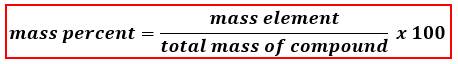# Problem: Calculate the percent composition by mass of each element in Al(OH)3. Use at least three significant figures.%  Al = %  O =%  H =

🤓 Based on our data, we think this question is relevant for Professor Overstreet's class at CSUF.

###### FREE Expert Solution

We are asked to calculate for the percent by mass (mass %) of each element in Al(OH)3 and to do that we’re going to use the mass percent formula shown below:First, we need to get the atomic weights of each element in Al(OH)3 using the periodic table and then calculate its molar mass.

Mass of Al:###### Problem Details

Calculate the percent composition by mass of each element in Al(OH)3. Use at least three significant figures.

%  Al =

%  O =

%  H =

What scientific concept do you need to know in order to solve this problem?

Our tutors have indicated that to solve this problem you will need to apply the Mass Percent Formula concept. You can view video lessons to learn Mass Percent Formula. Or if you need more Mass Percent Formula practice, you can also practice Mass Percent Formula practice problems.

What is the difficulty of this problem?

Our tutors rated the difficulty ofCalculate the percent composition by mass of each element in...as low difficulty.

How long does this problem take to solve?

Our expert Chemistry tutor, Dasha took 3 minutes and 23 seconds to solve this problem. You can follow their steps in the video explanation above.

What professor is this problem relevant for?

Based on our data, we think this problem is relevant for Professor Overstreet's class at CSUF.